Home | ARTS | Operations Management | Method of solution of a 2x2 zero-sum game without saddle point - Games With No Saddle Point

# Method of solution of a 2x2 zero-sum game without saddle point - Games With No Saddle Point

Posted On :  24.06.2018 10:41 pm

Suppose that a 2x2 game has no saddle point. Suppose the game has the following pay-off matrix.

Method of solution of a 2x2 zero-sum game without saddle point

Suppose that a 2x2 game has no saddle point. Suppose the game has the following pay-off matrix.Since this game has no saddle point, the following condition shall hold:

Max {Min {a , b}, Min {c , d }} Min {Max {a , c}, Max {b, d}}

In this case, the game is called a mixed game. No strategy of Player A can be called the best strategy for him. Therefore A has to use both of his strategies. Similarly no strategy of Player B can be called the best strategy for him and he has to use both of his strategies.

Let p be the probability that Player A will use his first strategy. Then the probability that Player A will use his second strategy is 1-p.

If Player B follows his first strategy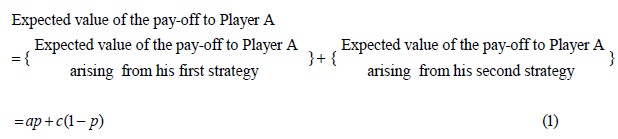In the above equation, note that the expected value is got as the product of the corresponding values of the pay-off and the probability.

If Player B follows his second strategy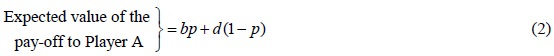If the expected values in equations (1) and (2) are different, Player B will prefer the minimum of the two expected values that he has to give to player A. Thus B will have a pure strategy. This contradicts our assumption that the game is a mixed one. Therefore the expected values of the pay-offs to Player A in equations (1) and (2) should be equal. Thus we have the conditionTo find the number of times that B will use his first strategy and second strategy:

Let the probability that B will use his first strategy be r. Then the probability that B will use his second strategy is 1-r.

When A use his first strategy

The expected value of loss to Player B with his first strategy = ar

The expected value of loss to Player B with his second strategy = b(1-r)

 Therefore the expected value of loss to B = ar + b(1-r) (3)

When A use his second strategy

The expected value of loss to Player B with his first strategy = cr

The expected value of loss to Player B with his second strategy = d(1-r)

 Therefore the expected value of loss to B = cr + d(1-r) (4)
If the two expected values are different then it results in a pure game, which is a contradiction. Therefore the expected values of loss to Player B in equations (3) and (4) should be equal. Hence we have the conditionProblem 2

Solve the following game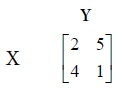Solution

First consider the row minima.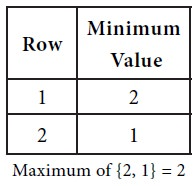Next consider the maximum of each column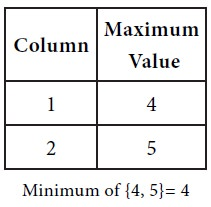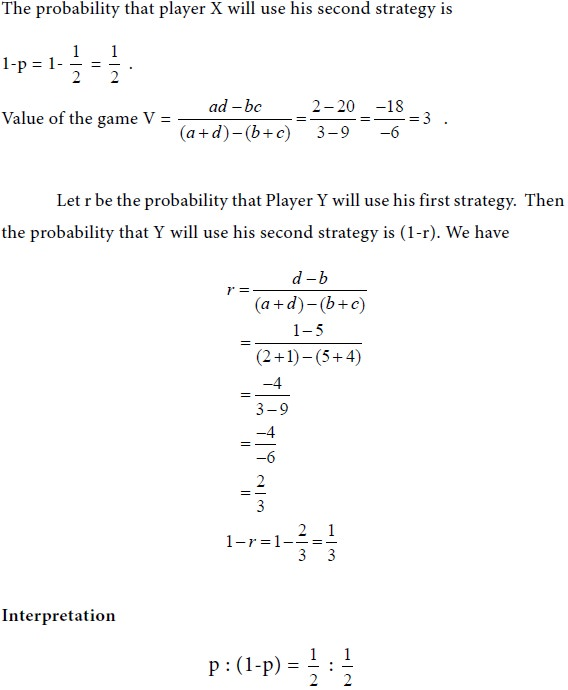Therefore, out of 2 trials, player X will use his first strategy once and his second strategy once.Therefore, out of 3 trials, player Y will use his first strategy twice and his second strategy once.

Tags : Operations Management - Game Theory, Goal Programming & Queuing Theory
Last 30 days 5755 views